Homework Help Question & Answers

# 3. Determine whether each of the following production functions below displays constant, increasing, or decreasing returns...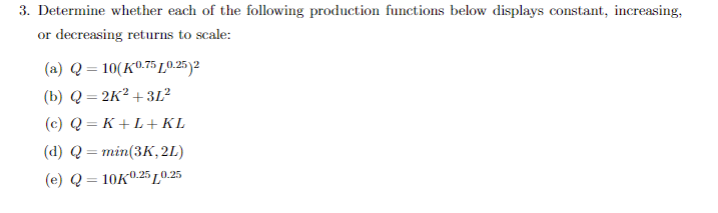3. Determine whether each of the following production functions below displays constant, increasing, or decreasing returns to scale: (a) Q = 10(K0.75 0.252 (b) Q = 2K2 +312 (c) Q=K+L+KL (d) Q = min(3K, 2L) (e) Q = 10K0:250.25

#### Homework Answers

Answer #1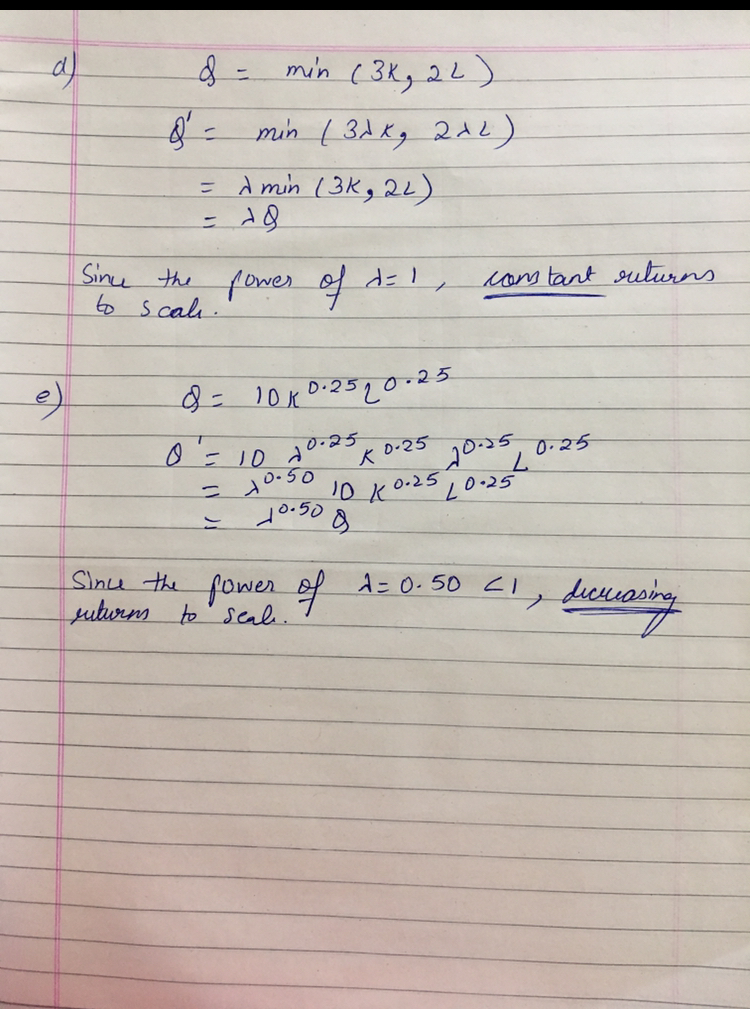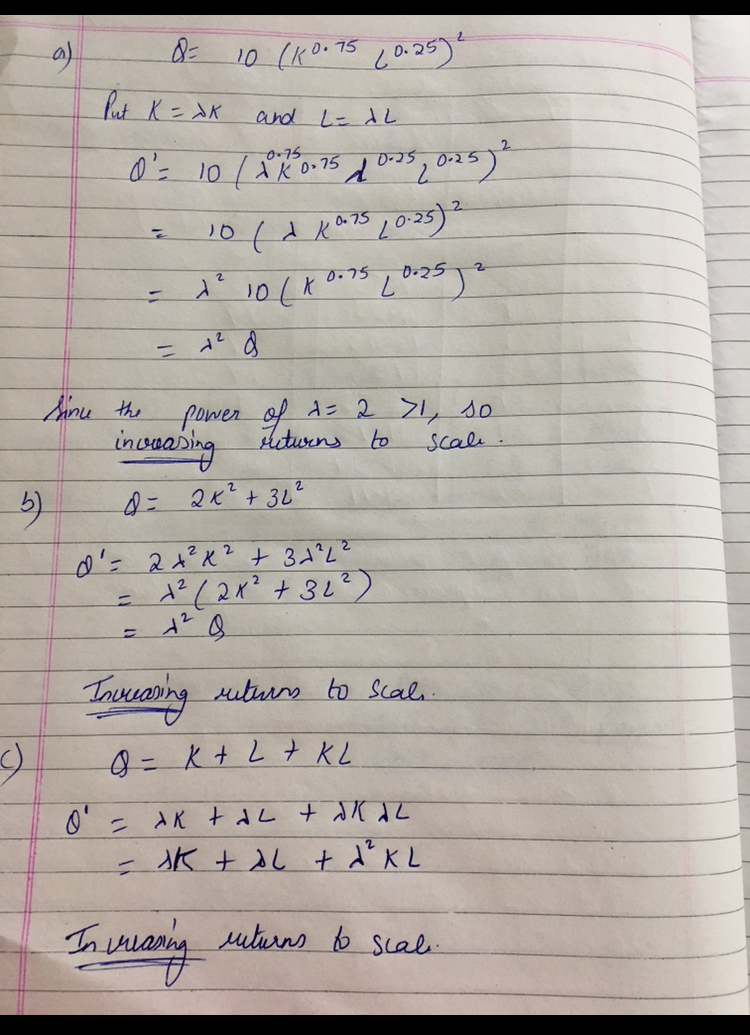Know the answer?
Your Answer:

#### Post as a guest

Your Name:

What's your source?

#### Earn Coin

Coins can be redeemed for fabulous gifts.

Not the answer you're looking for? Ask your own homework help question. Our experts will answer your question WITHIN MINUTES for Free.
Similar Homework Help Questions
• ### Determine whether each of the production functions below displays constant, increasing, or decreasing returns to scale:...

Determine whether each of the production functions below displays constant, increasing, or decreasing returns to scale: Q = K + L + KL Q = 2K2 + 3L2 Q = KL Q = min(3K, 2L)

• ### Determine whether each of the production functions below displays constant, increasing, or decreasing returns to scale:...

Determine whether each of the production functions below displays constant, increasing, or decreasing returns to scale: Q = 10K0.75L0.25 Q = (K0.75L0.25)2 Q = K 0.75L0.75 Q = K 0.25L0.25 Q = K + L + KL Q = 2K2 + 3L2 Q = KL Q = min(3K, 2L)

• ### 5. Determine whether each of the following production functions displays constant, increasing, or decreasing returns to...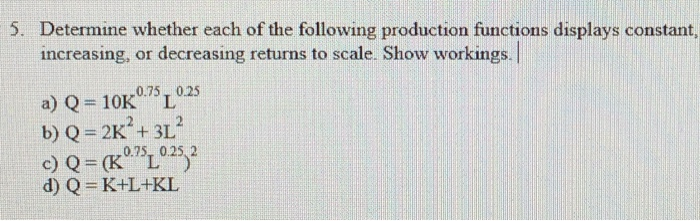5. Determine whether each of the following production functions displays constant, increasing, or decreasing returns to scale. Show workings. a) Q= 10K 0.75, 0.25 b) Q = 2K+ + 3L c) Q = (Kº75 0.25 2 d) Q=K+L+KL

• ### Briefly show whether the following production functions exhibit increasing, decreasing, or constant returns to scale: Y...

Briefly show whether the following production functions exhibit increasing, decreasing, or constant returns to scale: Y = K2/3 + L2/3 Y = min {2L+K, 2K+L} Y = 20*L1/5*K4/5

• ### 2) Determine whether the following production functions exhibit constant, increasing, or decreasing returns to scale (or...

2) Determine whether the following production functions exhibit constant, increasing, or decreasing returns to scale (or none of these) a) Y=K+L^1/3 b) Y= aln(L) + bIn(k)

• ### 1. Below are production functions that turn capital (K) and labor (L) into output. For each...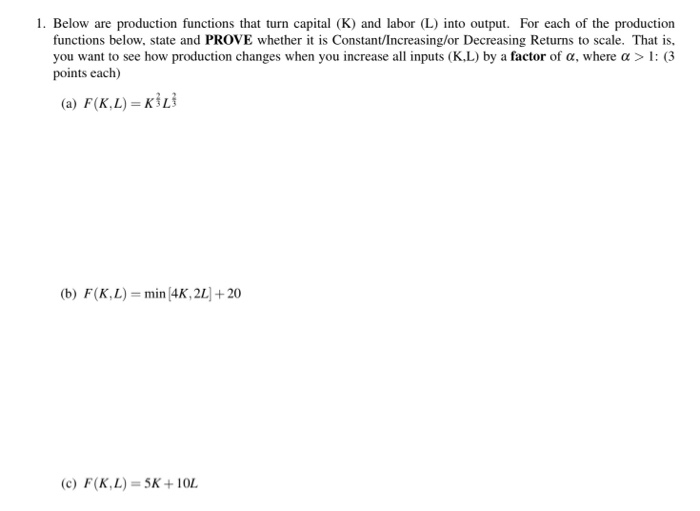1. Below are production functions that turn capital (K) and labor (L) into output. For each of the production functions below, state and PROVE whether it is Constant/Increasing/or Decreasing Returns to scale. That is, you want to see how production changes when you increase all inputs (KL) by a factor of a, where a > 1: (3 points each) (a) F(K.L) = (b) F(KL)= min (4K, 2L + 20 (c) F(K,L) = 5K+ 10L

• ### Added this question before but they were not correct, please double check answers. Thank you 5. Decide whether the following production functions belong to increasing, constant, or decreasing returns...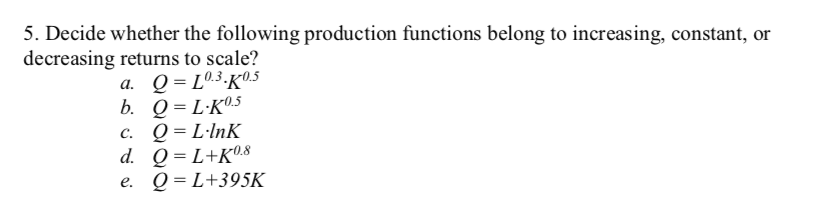Added this question before but they were not correct, please double check answers. Thank you 5. Decide whether the following production functions belong to increasing, constant, or decreasing returns to scale? Q = L+K's Q- L+395K d, e. 5. Decide whether the following production functions belong to increasing, constant, or decreasing returns to scale? Q = L+K's Q- L+395K d, e.

• ### 1. State whether the following production functions exhibit increasing, constant, or decreasing returns to scale in...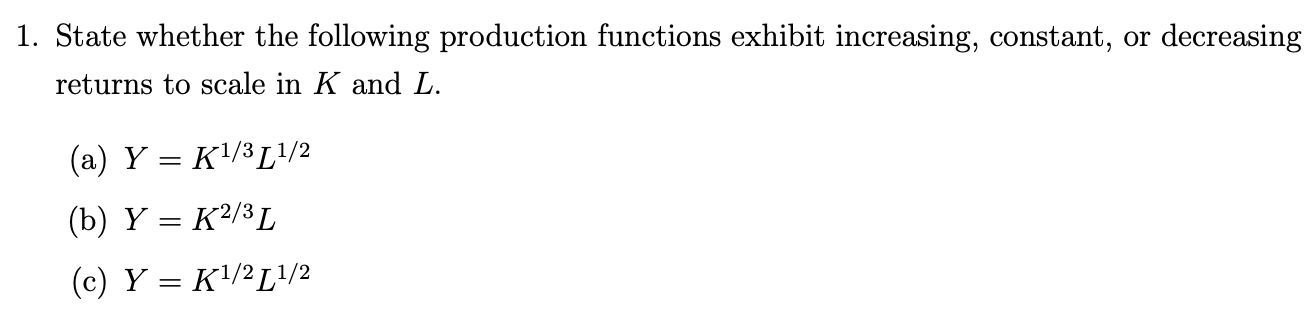1. State whether the following production functions exhibit increasing, constant, or decreasing returns to scale in K and L. (a) Y = K1/3L1/2 (b) Y = K2/3L (c) Y = K1/2 [1/2

• ### Q#02 Check whether the following production function exhibits (10 Marks) Constant Returns to Scale Increasing Returns...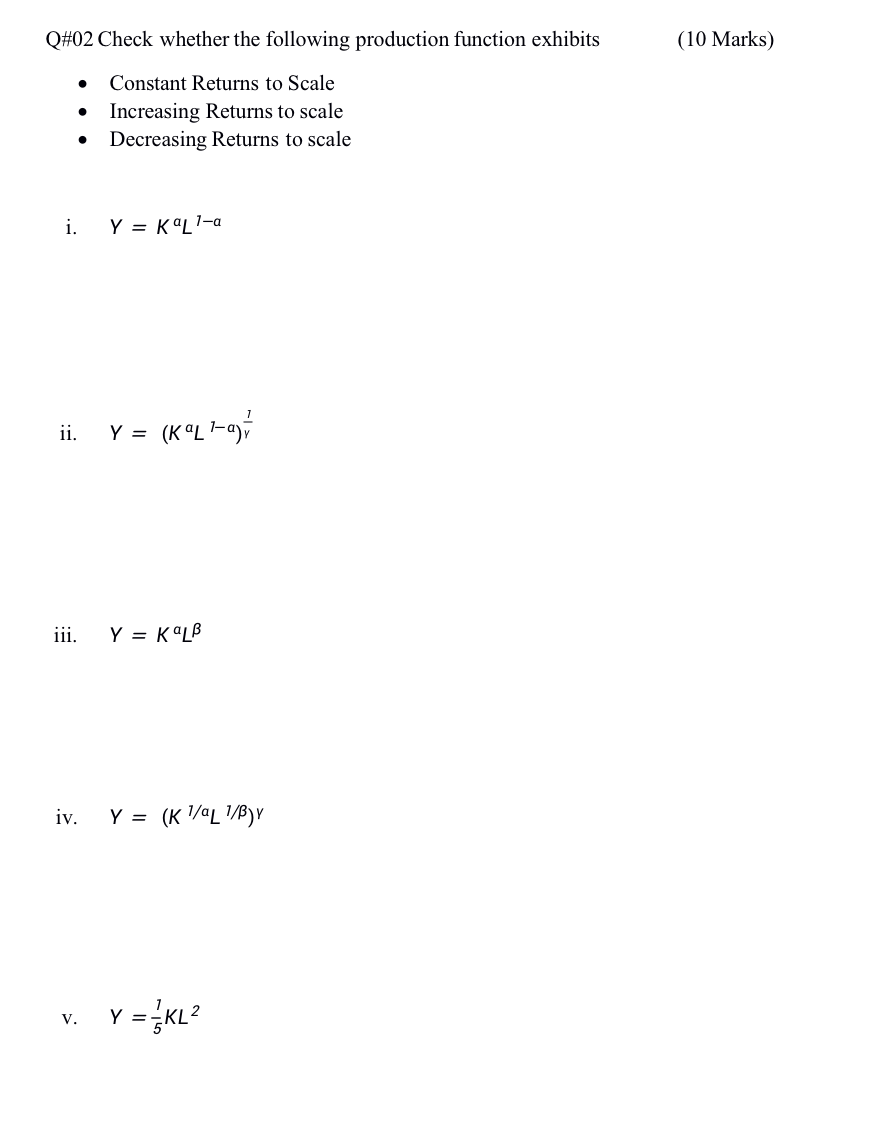Q#02 Check whether the following production function exhibits (10 Marks) Constant Returns to Scale Increasing Returns to scale Decreasing Returns to scale . i. Y = Kal1-a ii. Y = (KL-ay iii. Y = KOLB iv. Y = (K 1/4L 1/8), v. Y = KL

• ### 1. Below are production functions that turn capital (K) and labor (L) into output. For each...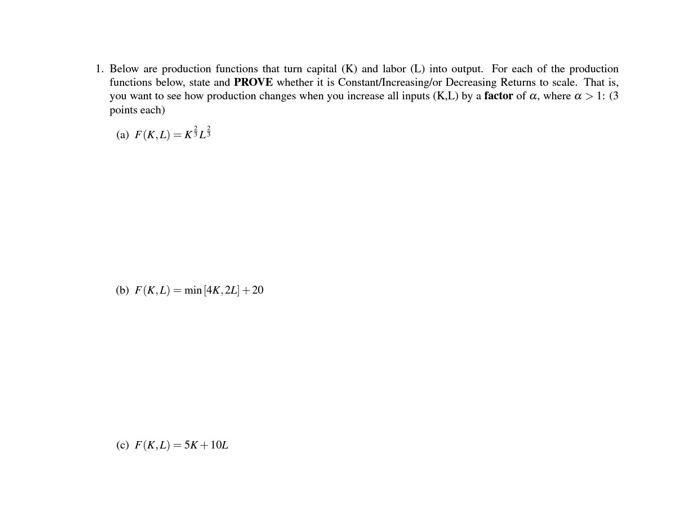1. Below are production functions that turn capital (K) and labor (L) into output. For each of the production functions below, state and PROVE whether it is Constant/Increasing/or Decreasing Returns to scale. That is, you want to see how production changes when you increase all inputs (K.L) by a factor of a, where a > 1: (3 points each) (a) F(KL)=KL (b) F(K,L) = min (4K, 22] + 20 (c) F(K,L) = 5K+10L

Free Homework App

Scan Your Homework
to Get Instant Free Answers
Need Online Homework Help?

Get Answers For Free
Most questions answered within 3 hours.